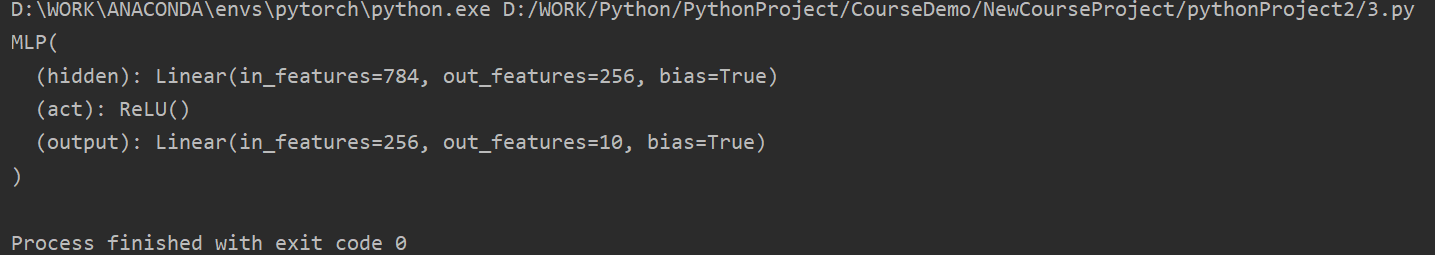###### Sleep1ng In
2022-06-24 11:16浏览 12

# Pycharm 中使用 net(X) 遇到的疑问

``````

import torch
from torch import nn

class MLP(nn.Module):

def __init__(self, **kwargs):

super(MLP, self).__init__(**kwargs)
self.hidden = nn.Linear(784, 256)
self.act = nn.ReLU()
self.output = nn.Linear(256, 10)

def forward(self, x):
a = self.act(self.hidden(x))
return self.output(a)
X = torch.rand(2, 784)
net = MLP()
print(net)
# print(net(X))
net(X)
``````